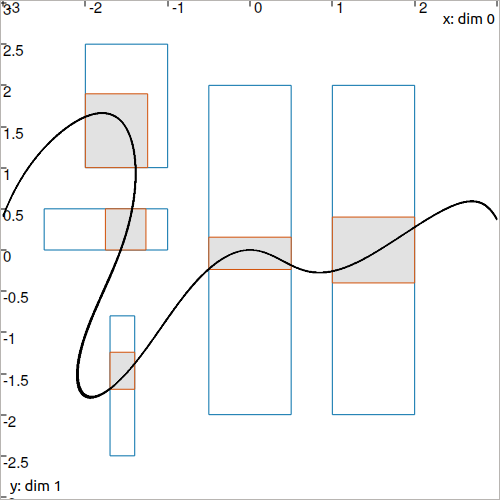# Generic CtcFunction: $$\mathbf{f}(\mathbf{x})=\mathbf{0}$$¶

Lot of constraints can be expressed under the form $$\mathbf{f}(\mathbf{x})=\mathbf{0}$$ with $$\mathbf{f}$$ an analytic function possibly non-linear. The goal is to estimate the set of feasible vectors $$\mathbf{x}$$ of a domain $$[\mathbf{x}]$$ that satisfy this constraint. A dedicated contractor can be built from $$\mathbf{f}$$ in order to contract boxes.

Note that the other form $$\mathbf{f}(\mathbf{x})\in[\mathbf{y}]$$ can also be treated by this contractor.

## Definition¶

Important

$\mathbf{f}(\mathbf{x})=\mathbf{0} \longrightarrow \mathcal{C}_{\mathbf{f}}\big([\mathbf{x}]\big) \mathrm{~~~~or~~~~} \mathbf{f}(\mathbf{x})\in[\mathbf{y}] \longrightarrow \mathcal{C}_{\mathbf{f},[\mathbf{y}]}\big([\mathbf{x}]\big)$
# For the constraint f(x)=0
ctc_f = CtcFunction(Function("<var1>", "<var2>", ..., "<expr>"))
ctc_f.contract(x)

# For the constraint f(x)ϵ[y]
y = Interval(...) # or IntervalVector if f is a vector function
ctc_f = CtcFunction(Function("<var1>", "<var2>", ..., "<expr>"), y)
ctc_f.contract(x)

// For the constraint f(x)=0
CtcFunction ctc_f(Function("<var1>", "<var2>", ..., "<expr>"));
ctc_f.contract(x);

// For the constraint f(x)ϵ[y]
Interval y(...); // or IntervalVector if f is a vector function
CtcFunction ctc_f(Function("<var1>", "<var2>", ..., "<expr>"), y);
ctc_f.contract(x);


NoteThis contractor originates from the IBEX library. It is briefly presented here for the sake of consistency. For more information, please refer to the IBEX documentation for C++.

Optimality

This contractor is not necessarily optimal, depending on the expression of $$\mathbf{f}$$.

## Example¶

The constraint:

$x_1+x_2=x_3$

is equivalent to:

$f(\mathbf{x})=0 \mathrm{~~~with~~~} f(\mathbf{x})=x_1+x_2-x_3$

We can then build the contractor with:

ctc_f = CtcFunction(Function("x1", "x2", "x3", "x1+x2-x3"))

CtcFunction ctc_f(Function("x1", "x2", "x3", "x1+x2-x3"));


## Another example¶

Let us consider the following non-linear function:

$f(\mathbf{x}) = x_1\cos(x_1-x_2)\sin(x_1)+x_2$

A contractor for the constraint $$f(\mathbf{x})=0$$ can be built by:

ctc_f = CtcFunction(Function("x1", "x2", "x1*cos(x1-x2)*sin(x1)+x2"))

CtcFunction ctc_f(Function("x1", "x2", "x1*cos(x1-x2)*sin(x1)+x2"));

The first parameters are the variables names. The last one is the expression of $$f$$.
Note that it is also possible to write vector variables:
ctc_f = CtcFunction(Function("x", "x*cos(x-x)*sin(x)+x"))

CtcFunction ctc_f(Function("x", "x*cos(x-x)*sin(x)+x"));


Then, a box $$[\mathbf{x}]$$ can be contracted by:

x = IntervalVector([[-2,-1],[1,2.5]])
ctc_f.contract(x)

IntervalVector x({{-2.,-1.},{1.,2.5}});
ctc_f.contract(x);


The boxes are contracted in order to remove some vectors that are not consistent with $$f(\mathbf{x})=0$$. In the following figure, the exact solution for $$f(\mathbf{x})=0$$ is black painted. The initial boxes are depicted in blue, their contraction is represented in red.Fig. 15 Illustration of several contracted boxes with the above ctc_f contractor.

## Dealing with inequalities¶

For constraints under the form $$\mathbf{f}(\mathbf{x})\in[\mathbf{y}]$$ (instead of $$\mathbf{f}(\mathbf{x})=\mathbf{0}$$ as in the previous section), one can specify $$[\mathbf{y}]$$ as last optional argument of CtcFunction.

A constraint $$\mathbf{f}(\mathbf{x})\leqslant 0$$ is equivalent to $$\mathbf{f}(\mathbf{x})\in[-\infty,0]$$ and so the related contractor becomes:

ctc_f = CtcFunction(Function("x", "x*cos(x-x)*sin(x)+x"), Interval(-oo,0))

CtcFunction ctc_f(Function("x", "x*cos(x-x)*sin(x)+x"), Interval(-oo,0));


The above illustration reveals several contracted boxes with the new ctc_f contractor, in the case of inequalities. The actual solution set $$\mathbb{X}=\{\mathbf{x}\mid\mathbf{f}(\mathbf{x})\leqslant 0\}$$ is green painted.

## Going further¶

This CtcFunction class is a generic shortcut to deal with $$\mathbf{f}(\mathbf{x})=\mathbf{0}$$ or $$\mathbf{f}(\mathbf{x})\in[\mathbf{y}]$$. However, several algorithms exist to optimally deal with different classes of problems. A list of static contractors is provided in the IBEX library: see more. The user is invited to use an appropriate tool to deal with the constraint at stake.

The IBEX contractor behind CtcFunction is a CtcFwdBwd coupled with a Ctc3BCid.

Technical documentation